# 【恋上数据结构】冒泡排序、选择排序、堆排序

### 冒泡、选择、堆排序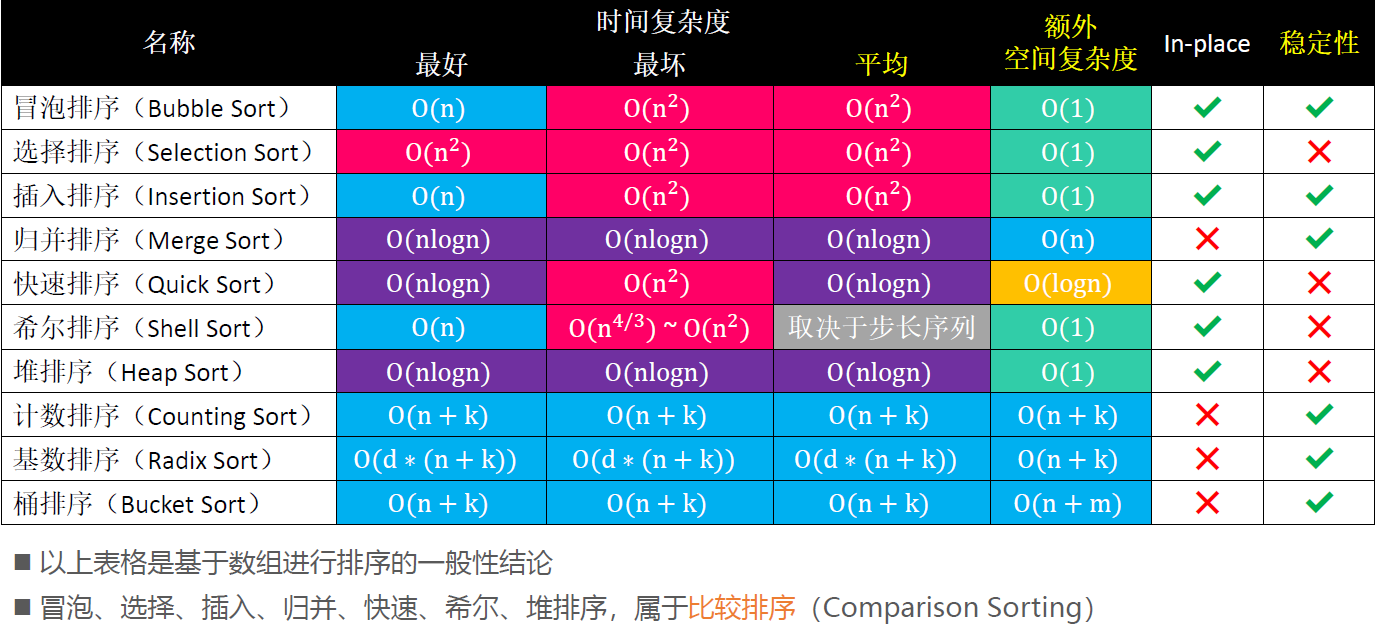# 冒泡排序

• ① 从头开始比较每一对相邻元素，如果第1个比第2个大，就交换它们的位置
执行完一轮后，最未尾那个元素就是最大的元素。
• ② 忽略①中曾经找到的最大元素，重复执行步骤①，直到全部元素有序。

/**
* 冒泡排序-无优化
*/
public class BubbleSort1<T extends Comparable<T>> extends Sort<T>{

@Override
protected void sort() {
for (int end = array.length - 1; end > 0; end--) {
for (int begin = 1; begin <= end; begin++) {
if (cmp(begin, begin - 1) < 0) {
swap(begin, begin - 1);
}
}
}
}

}


## 冒泡排序-优化1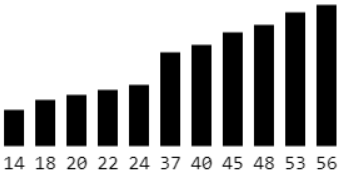/**
* 冒泡排序-优化1
* 如果序列已经完全有序，可以提前终止冒泡排序
*/
public class BubbleSort2<T extends Comparable<T>> extends Sort<T>{

@Override
protected void sort() {
for (int end = array.length - 1; end > 0; end--) {
boolean sorted = true;
for (int begin = 1; begin <= end; begin++) {
if (cmp(begin, begin - 1) < 0) {
swap(begin, begin - 1);
sorted = false;
}
}
if (sorted) break;
}
}

}


## 冒泡排序-优化2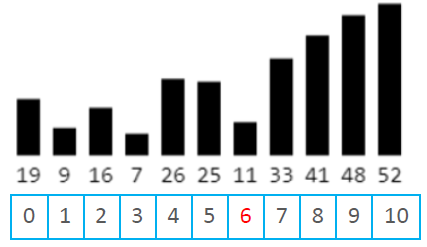/**
* 冒泡排序-优化2
* 如果序列尾部已经局部有序，可以记录最后1次交换的位置，减少比较次数
*/
public class BubbleSort3<T extends Comparable<T>> extends Sort<T>{

@Override
protected void sort() {
for (int end = array.length - 1; end > 0; end--) {
// 设为1是因为:如果这轮遍历一次交换都没进行
// 则说明序列后面已经有序, 则 end = sortedIndex = 1
// end--后等于0,会直接跳出循环
int sortedIndex = 1;
for (int begin = 1; begin <= end; begin++) {
if (cmp(begin, begin - 1) < 0) {
swap(begin, begin - 1);
// 记录最后1次交换的位置，减少比较次数
sortedIndex = begin;
}
}
end = sortedIndex;
}
}

}


## 复杂度与稳定性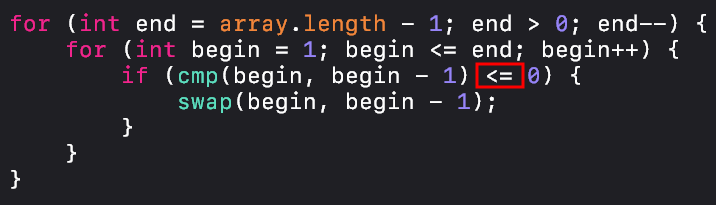# 选择排序

• ① 从序列中找出最大的那个元素，然后与最未尾的元素交换位置
执行完一轮后，最未尾的那个元素就是最大的元素。
• ② 忽略①中曾经找到的最大元素，重复执行步骤①。
/**
* 选择排序
*/
public class SelectionSort<T extends Comparable<T>> extends Sort<T> {

@Override
protected void sort() {
for (int end = array.length - 1; end > 0; end--) {
int max = 0;
for (int begin = 1; begin <= end; begin++) {
if (cmp(max, begin) < 0) {
max = begin;
}
}
swap(max, end);
}
}

}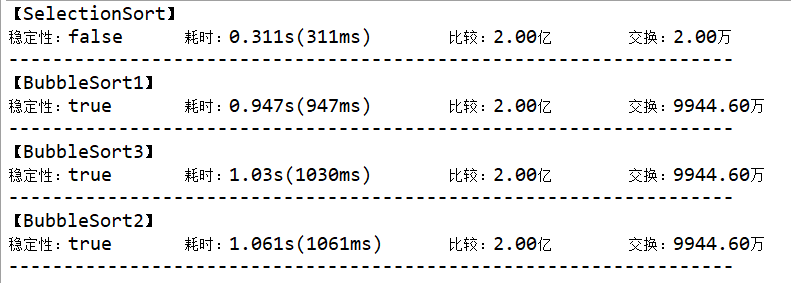## 复杂度与稳定性

• 最好、最坏、平均时间复杂度：$O(n^2)$
• 空间复杂度：$O(1)$
• 选择排序属于不稳定排序

• 使用堆来选择最大值，可以大大提高选择速度

# 堆排序

• ① 对序列进行原地建堆（heapify）
• ② 重复执行以下操作（依次取出堆中最大值，并删除），直到堆的元素数量为 1
• 交换堆顶元素与堆尾元素（把最大值放到最后面）
• 堆的元素数量减 1（不管最后已经放到最后的最大值）
• 对 0 位置进行 1 次 siftDown 操作

## 堆排序图解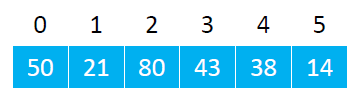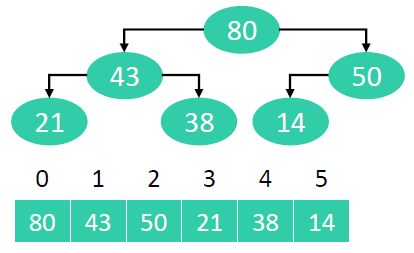• 交换堆顶元素与尾元素
• 堆的元素数量减 1
• 对 0 位置进行 1 次 siftDown 操作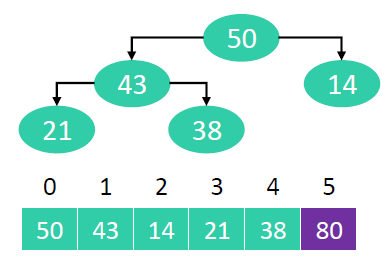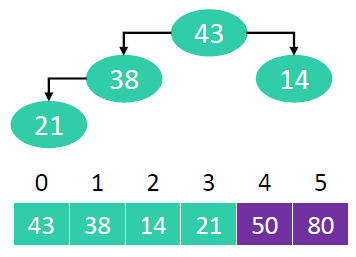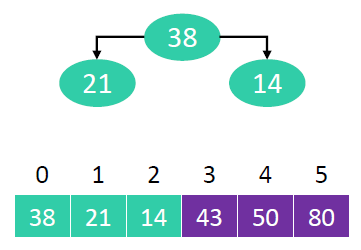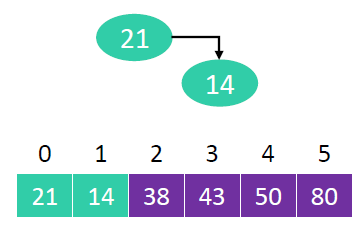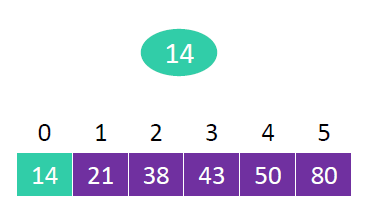## 堆排序实现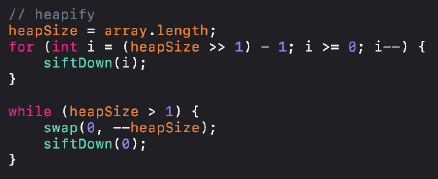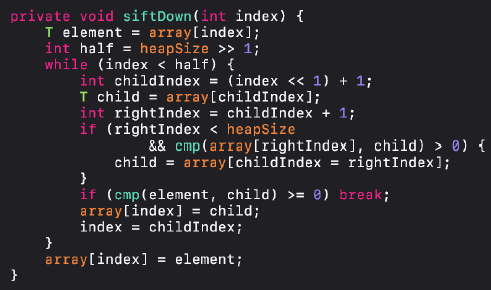/**
* 堆排序
*/
public class HeapSort<T extends Comparable<T>> extends Sort<T> {
private int heapSize; // 堆大小

@Override
protected void sort() {
// 原地建堆（自下而上的下滤）
heapSize = array.length;
for (int i = (heapSize >> 1) - 1; i >= 0; i--) {
siftDown(i);
}

while (heapSize > 1) {
// 交换堆顶元素和尾部元素
swap(0, --heapSize);

// 对0位置进行siftDown（恢复堆的性质）
siftDown(0);
}
}

private void siftDown(int index) {
T element = array[index];

int half = heapSize >> 1;
while (index < half) { // index必须是非叶子节点
// 默认是左边跟父节点比
int childIndex = (index << 1) + 1;
T child = array[childIndex];

int rightIndex = childIndex + 1;
// 右子节点要存在, 并且比左子节点大
if (rightIndex < heapSize &&
cmp(array[rightIndex], child) > 0) {
child = array[childIndex = rightIndex];
}

// 大于等于子节点
if (cmp(element, child) >= 0) break;

array[index] = child;
index = childIndex;
}
array[index] = element;
}

}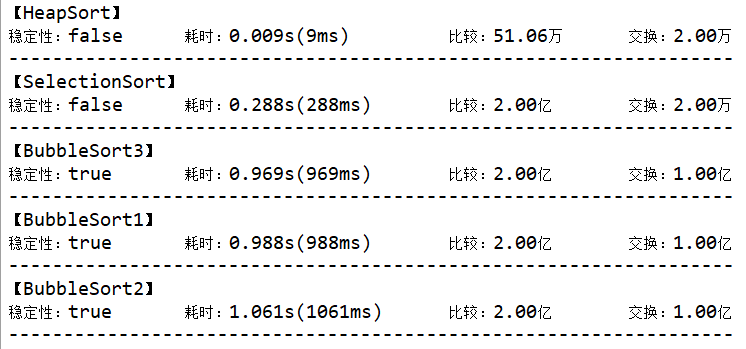## 复杂度与稳定性

• 最好、最坏、平均时间复杂度：$O(nlogn)$
• 空间复杂度：$O(1)$
• 堆排序属于不稳定排序©️2019 CSDN 皮肤主题: 黑客帝国 设计师: 上身试试